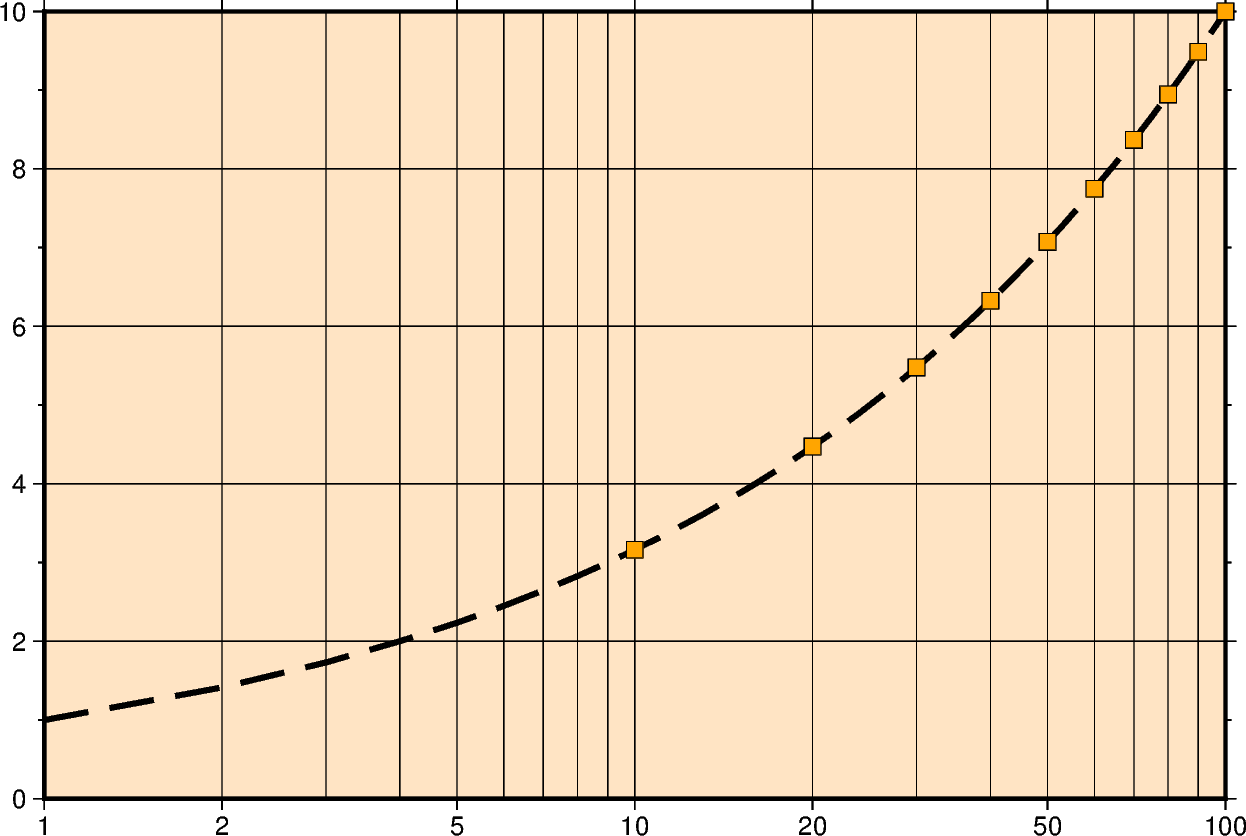# Cartesian logarithmic

Xwidth[l]/[height[l]]: Give the width of the figure and the optional height. The axis or axes with a logarithmic transformation requires l after its size argument.Out:

```<IPython.core.display.Image object>
```

```import numpy as np
import pygmt

# Create a list of x values 0-100
xline = np.arange(0, 101)
# Create a list of y-values that are the square root of the x-values
yline = xline**0.5
# Create a list of x values for every 10 in 0-100
xpoints = np.arange(0, 101, 10)
# Create a list of y-values that are the square root of the x-values
ypoints = xpoints**0.5

fig = pygmt.Figure()
fig.plot(
region=[1, 100, 0, 10],
# Set a logarithmic transformation on the x-axis
projection="X15cl/10c",
# Set the figures frame, color, and gridlines
frame=["WSne+gbisque", "x2g3", "ya2f1g2"],
x=xline,
y=yline,
# Set the line thickness to *1p*, the color to *blue*, and the style to
# *dash*
pen="1p,blue,-",
)
# Plot square root values as points on the line
# Style of points is 0.3 cm square, color is *red* with a *black* outline
# Points are not clipped if they go off the figure
fig.plot(x=xpoints, y=ypoints, style="s0.3c", color="red", no_clip=True, pen="black")
fig.show()
```

Total running time of the script: ( 0 minutes 0.963 seconds)

Gallery generated by Sphinx-Gallery